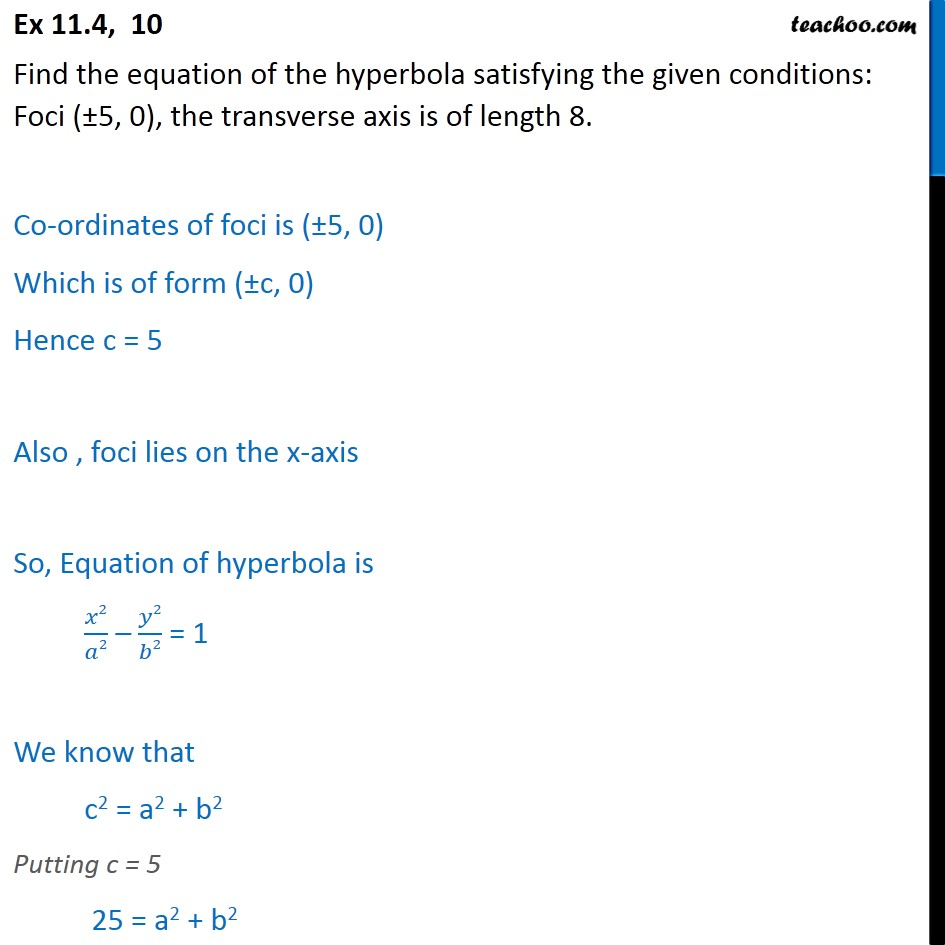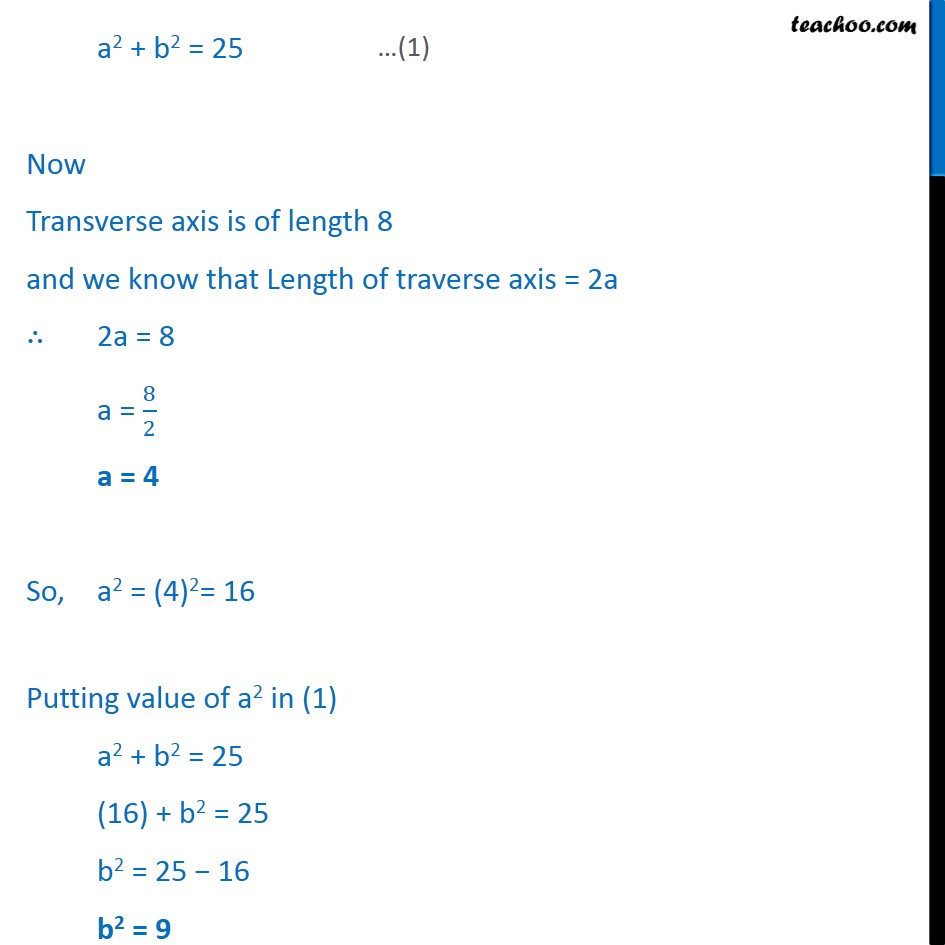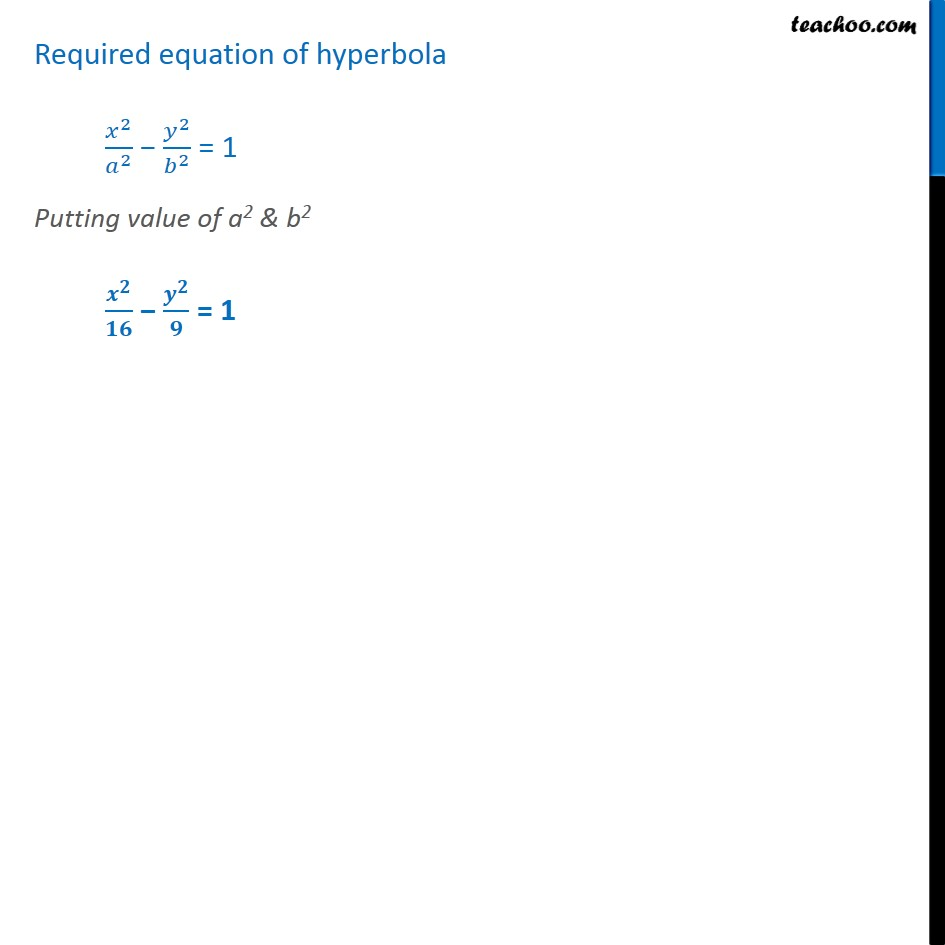Ex 10.4

Chapter 10 Class 11 Conic Sections
Serial order wiseLearn in your speed, with individual attention - Teachoo Maths 1-on-1 Class

### Transcript

Ex 10.4, 10 Find the equation of the hyperbola satisfying the given conditions: Foci ( 5, 0), the transverse axis is of length 8. Co-ordinates of foci is ( 5, 0) Which is of form ( c, 0) Hence c = 5 Also , foci lies on the x-axis So, Equation of hyperbola is 2 2 2 2 = 1 We know that c2 = a2 + b2 Putting c = 5 25 = a2 + b2 a2 + b2 = 25 Now Transverse axis is of length 8 and we know that Length of traverse axis = 2a 2a = 8 a = 8 2 a = 4 So, a2 = (4)2= 16 Putting value of a2 in (1) a2 + b2 = 25 (16) + b2 = 25 b2 = 25 16 b2 = 9 Required equation of hyperbola 2 2 2 2 = 1 Putting value of a2 & b2 = 1